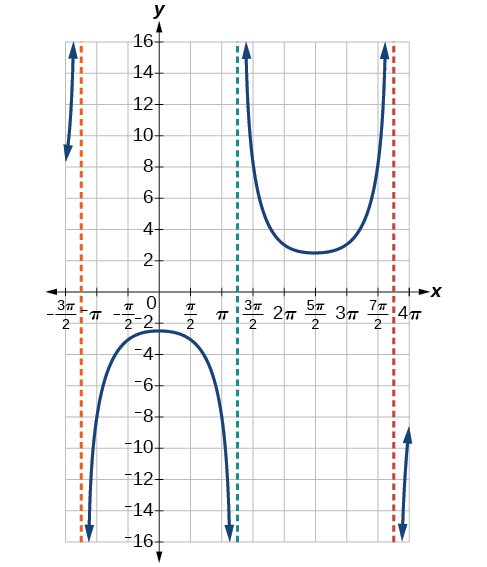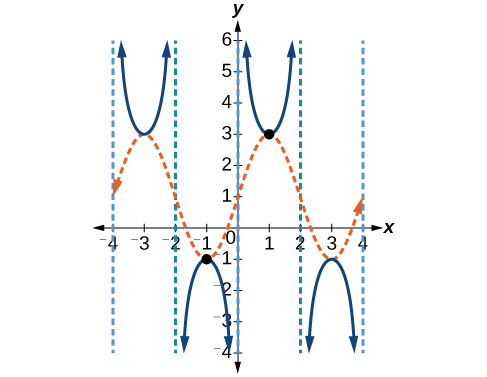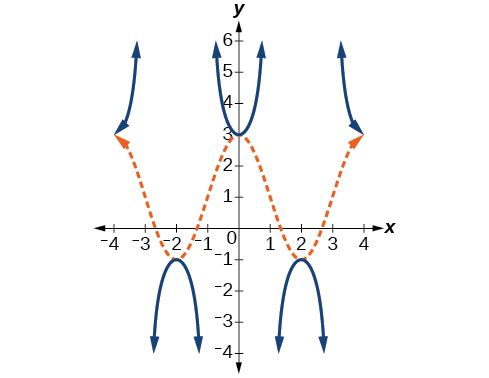# 2.2 Graphs of the other trigonometric functions  (Page 6/9)

 Page 6 / 9

The domain of $\text{\hspace{0.17em}}\mathrm{csc}\text{\hspace{0.17em}}x\text{\hspace{0.17em}}$ was given to be all $\text{\hspace{0.17em}}x\text{\hspace{0.17em}}$ such that $\text{\hspace{0.17em}}x\ne k\pi \text{\hspace{0.17em}}$ for any integer $\text{\hspace{0.17em}}k.\text{\hspace{0.17em}}$ Would the domain of $\text{\hspace{0.17em}}y=A\mathrm{csc}\left(Bx-C\right)+D\text{\hspace{0.17em}}\text{be}\text{\hspace{0.17em}}x\ne \frac{C+k\pi }{B}?$

Yes. The excluded points of the domain follow the vertical asymptotes. Their locations show the horizontal shift and compression or expansion implied by the transformation to the original function’s input.

Given a function of the form $\text{\hspace{0.17em}}y=A\mathrm{csc}\left(Bx\right),\text{\hspace{0.17em}}$ graph one period.

1. Express the function given in the form $\text{\hspace{0.17em}}y=A\mathrm{csc}\left(Bx\right).$
2. $\text{\hspace{0.17em}}|A|.$
3. Identify $\text{\hspace{0.17em}}B\text{\hspace{0.17em}}$ and determine the period, $\text{\hspace{0.17em}}P=\frac{2\pi }{|B|}.$
4. Draw the graph of $\text{\hspace{0.17em}}y=A\mathrm{sin}\left(Bx\right).$
5. Use the reciprocal relationship between $\text{\hspace{0.17em}}y=\mathrm{sin}\text{\hspace{0.17em}}x\text{\hspace{0.17em}}$ and $\text{\hspace{0.17em}}y=\mathrm{csc}\text{\hspace{0.17em}}x\text{\hspace{0.17em}}$ to draw the graph of $\text{\hspace{0.17em}}y=A\mathrm{csc}\left(Bx\right).$
6. Sketch the asymptotes.
7. Plot any two reference points and draw the graph through these points.

## Graphing a variation of the cosecant function

Graph one period of $\text{\hspace{0.17em}}f\left(x\right)=-3\mathrm{csc}\left(4x\right).$

• Step 1. The given function is already written in the general form, $\text{\hspace{0.17em}}y=A\mathrm{csc}\left(Bx\right).$
• Step 2. $\text{\hspace{0.17em}}|A|=|-3|=3,$ so the stretching factor is 3.
• Step 3. $\text{\hspace{0.17em}}B=4,$ so $\text{\hspace{0.17em}}P=\frac{2\pi }{4}=\frac{\pi }{2}.\text{\hspace{0.17em}}$ The period is $\text{\hspace{0.17em}}\frac{\pi }{2}\text{\hspace{0.17em}}$ units.
• Step 4. Sketch the graph of the function $\text{\hspace{0.17em}}g\left(x\right)=-3\mathrm{sin}\left(4x\right).$
• Step 5. Use the reciprocal relationship of the sine and cosecant functions to draw the cosecant function .
• Steps 6–7. Sketch three asymptotes at $\text{\hspace{0.17em}}x=0,\text{\hspace{0.17em}}x=\frac{\pi }{4},\text{\hspace{0.17em}}$ and $\text{\hspace{0.17em}}x=\frac{\pi }{2}.\text{\hspace{0.17em}}$ We can use two reference points, the local maximum at $\text{\hspace{0.17em}}\left(\frac{\pi }{8},-3\right)\text{\hspace{0.17em}}$ and the local minimum at $\text{\hspace{0.17em}}\left(\frac{3\pi }{8},3\right).$ [link] shows the graph.

Graph one period of $\text{\hspace{0.17em}}f\left(x\right)=0.5\mathrm{csc}\left(2x\right).$Given a function of the form $\text{\hspace{0.17em}}f\left(x\right)=A\mathrm{csc}\left(Bx-C\right)+D,\text{\hspace{0.17em}}$ graph one period.

1. Express the function given in the form $\text{\hspace{0.17em}}y=A\mathrm{csc}\left(Bx-C\right)+D.$
2. Identify the stretching/compressing factor, $\text{\hspace{0.17em}}|A|.$
3. Identify $\text{\hspace{0.17em}}B\text{\hspace{0.17em}}$ and determine the period, $\text{\hspace{0.17em}}\frac{2\pi }{|B|}.$
4. Identify $\text{\hspace{0.17em}}C\text{\hspace{0.17em}}$ and determine the phase shift, $\text{\hspace{0.17em}}\frac{C}{B}.$
5. Draw the graph of $\text{\hspace{0.17em}}y=A\mathrm{csc}\left(Bx\right)\text{\hspace{0.17em}}$ but shift it to the right by and up by $\text{\hspace{0.17em}}D.$
6. Sketch the vertical asymptotes, which occur at $\text{\hspace{0.17em}}x=\frac{C}{B}+\frac{\pi }{|B|}k,$ where $\text{\hspace{0.17em}}k\text{\hspace{0.17em}}$ is an integer.

## Graphing a vertically stretched, horizontally compressed, and vertically shifted cosecant

Sketch a graph of $\text{\hspace{0.17em}}y=2\mathrm{csc}\left(\frac{\pi }{2}x\right)+1.\text{\hspace{0.17em}}$ What are the domain and range of this function?

• Step 1. Express the function given in the form $\text{\hspace{0.17em}}y=2\mathrm{csc}\left(\frac{\pi }{2}x\right)+1.$
• Step 2. Identify the stretching/compressing factor, $\text{\hspace{0.17em}}|A|=2.$
• Step 3. The period is $\text{\hspace{0.17em}}\frac{2\pi }{|B|}=\frac{2\pi }{\frac{\pi }{2}}=\frac{2\pi }{1}\cdot \frac{2}{\pi }=4.$
• Step 4. The phase shift is $\text{\hspace{0.17em}}\frac{0}{\frac{\pi }{2}}=0.$
• Step 5. Draw the graph of $\text{\hspace{0.17em}}y=A\mathrm{csc}\left(Bx\right)\text{\hspace{0.17em}}$ but shift it up $\text{\hspace{0.17em}}D=1.$
• Step 6. Sketch the vertical asymptotes, which occur at $\text{\hspace{0.17em}}x=0,x=2,x=4.$

The graph for this function is shown in [link] .A transformed cosecant function

Given the graph of $\text{\hspace{0.17em}}f\left(x\right)=2\mathrm{cos}\left(\frac{\pi }{2}x\right)+1\text{\hspace{0.17em}}$ shown in [link] , sketch the graph of $\text{\hspace{0.17em}}g\left(x\right)=2\mathrm{sec}\left(\frac{\pi }{2}x\right)+1\text{\hspace{0.17em}}$ on the same axes.## Analyzing the graph of y = cot x

The last trigonometric function we need to explore is cotangent    . The cotangent is defined by the reciprocal identity $\text{\hspace{0.17em}}\mathrm{cot}\text{\hspace{0.17em}}x=\frac{1}{\mathrm{tan}\text{\hspace{0.17em}}x}.\text{\hspace{0.17em}}$ Notice that the function is undefined when the tangent function is 0, leading to a vertical asymptote in the graph at $\text{\hspace{0.17em}}0,\pi ,\text{\hspace{0.17em}}$ etc. Since the output of the tangent function is all real numbers, the output of the cotangent function is also all real numbers.

We can graph $\text{\hspace{0.17em}}y=\mathrm{cot}\text{\hspace{0.17em}}x\text{\hspace{0.17em}}$ by observing the graph of the tangent function because these two functions are reciprocals of one another. See [link] . Where the graph of the tangent function decreases, the graph of the cotangent function increases. Where the graph of the tangent function increases, the graph of the cotangent function decreases.

#### Questions & Answers

how can chip be made from sand
Eke Reply
is this allso about nanoscale material
Almas
are nano particles real
Missy Reply
yeah
Joseph
Hello, if I study Physics teacher in bachelor, can I study Nanotechnology in master?
Lale Reply
no can't
Lohitha
where is the latest information on a no technology how can I find it
William
currently
William
where we get a research paper on Nano chemistry....?
Maira Reply
nanopartical of organic/inorganic / physical chemistry , pdf / thesis / review
Ali
what are the products of Nano chemistry?
Maira Reply
There are lots of products of nano chemistry... Like nano coatings.....carbon fiber.. And lots of others..
learn
Even nanotechnology is pretty much all about chemistry... Its the chemistry on quantum or atomic level
learn
Google
da
no nanotechnology is also a part of physics and maths it requires angle formulas and some pressure regarding concepts
Bhagvanji
hey
Giriraj
Preparation and Applications of Nanomaterial for Drug Delivery
Hafiz Reply
revolt
da
Application of nanotechnology in medicine
has a lot of application modern world
Kamaluddeen
yes
narayan
what is variations in raman spectra for nanomaterials
Jyoti Reply
ya I also want to know the raman spectra
Bhagvanji
I only see partial conversation and what's the question here!
Crow Reply
what about nanotechnology for water purification
RAW Reply
please someone correct me if I'm wrong but I think one can use nanoparticles, specially silver nanoparticles for water treatment.
Damian
yes that's correct
Professor
I think
Professor
Nasa has use it in the 60's, copper as water purification in the moon travel.
Alexandre
nanocopper obvius
Alexandre
what is the stm
Brian Reply
is there industrial application of fullrenes. What is the method to prepare fullrene on large scale.?
Rafiq
industrial application...? mmm I think on the medical side as drug carrier, but you should go deeper on your research, I may be wrong
Damian
How we are making nano material?
LITNING Reply
what is a peer
LITNING Reply
What is meant by 'nano scale'?
LITNING Reply
What is STMs full form?
LITNING
scanning tunneling microscope
Sahil
how nano science is used for hydrophobicity
Santosh
Do u think that Graphene and Fullrene fiber can be used to make Air Plane body structure the lightest and strongest. Rafiq
Rafiq
what is differents between GO and RGO?
Mahi
what is simplest way to understand the applications of nano robots used to detect the cancer affected cell of human body.? How this robot is carried to required site of body cell.? what will be the carrier material and how can be detected that correct delivery of drug is done Rafiq
Rafiq
if virus is killing to make ARTIFICIAL DNA OF GRAPHENE FOR KILLED THE VIRUS .THIS IS OUR ASSUMPTION
Anam
analytical skills graphene is prepared to kill any type viruses .
Anam
Any one who tell me about Preparation and application of Nanomaterial for drug Delivery
Hafiz
what is Nano technology ?
Bob Reply
write examples of Nano molecule?
Bob
The nanotechnology is as new science, to scale nanometric
brayan
nanotechnology is the study, desing, synthesis, manipulation and application of materials and functional systems through control of matter at nanoscale
Damian
Got questions? Join the online conversation and get instant answers!
Jobilize.com Reply

### Read also:

#### Get Jobilize Job Search Mobile App in your pocket Now!

Source:  OpenStax, Essential precalculus, part 2. OpenStax CNX. Aug 20, 2015 Download for free at http://legacy.cnx.org/content/col11845/1.2
Google Play and the Google Play logo are trademarks of Google Inc.

Notification Switch

Would you like to follow the 'Essential precalculus, part 2' conversation and receive update notifications?By Dionne MahaffeyBy Jonathan LongByBy Sandhills MLTBy Steve GibbsBy OpenStaxBy Nicole DuquetteBy Anh DaoBy Frank LevyBy Saylor Foundation